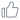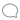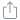# 面试官：来讲一讲进程

linux 进程如何创建

Linux系统创建进程都是由已存在的进程创建的（除了0号进程），被创建的进程叫做子进程，创建子进程的进程就做父进程。这句话是不是有点熟悉，没错，Linux进程串起来也是一颗树的结构```#include<stdio.h>
#include<unistd.h>

int main()
{
int p_num = 0;
int c_num = 0;
int pid = fork();
if(pid == 0) //返回的pid为0为子进程
{
c_num++;
}
else
{
p_num++; //返回的pid大于0为父进程
}
printf("p_num=%d, c_num=%d\n",p_num,c_num);
printf("pid=%d\n",pid);
return 0;
}
//运行结果如下所示
p_num=1, c_num=0
pid=36101
p_num=0, c_num=1
pid=0
``````//process.c
#include<stdio.h>
#include<unistd.h>

int main()
{
int pid = fork();
if(pid == 0)
{
execv("./test.o",NULL);  //test.o是一个经过编译的c语言文件，这里记得要放test.o的绝对路径
}
printf("This is parent process\n");
return 0;
}

//test.c
#include<stdio.h>
int main()
{
printf("This is child process");
return 0;
}

//运行结果如下所示
This is parent process
This is child process
```10分享